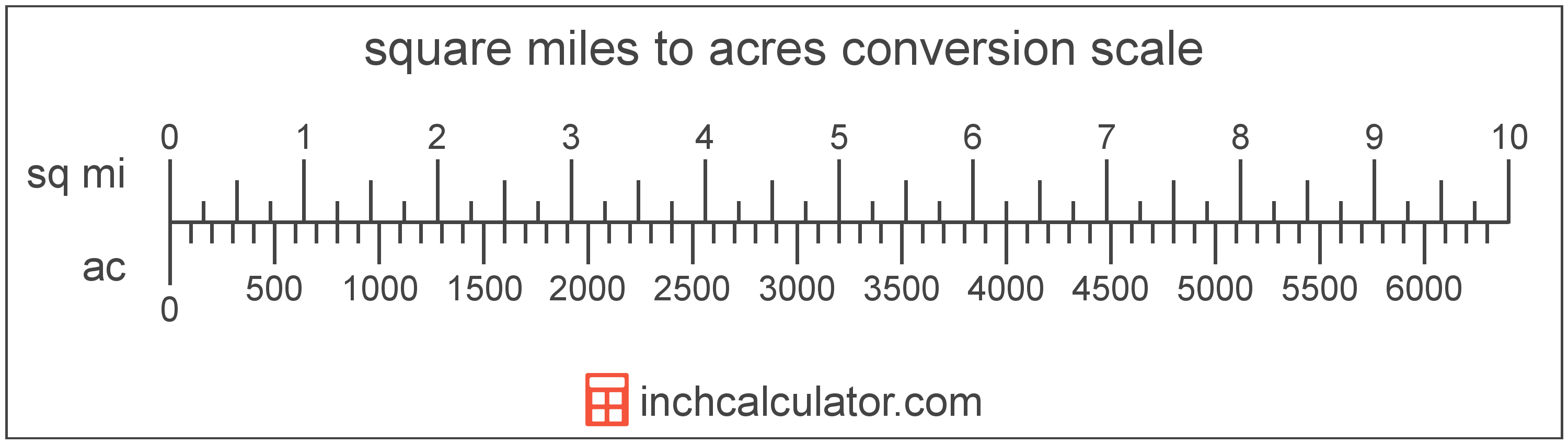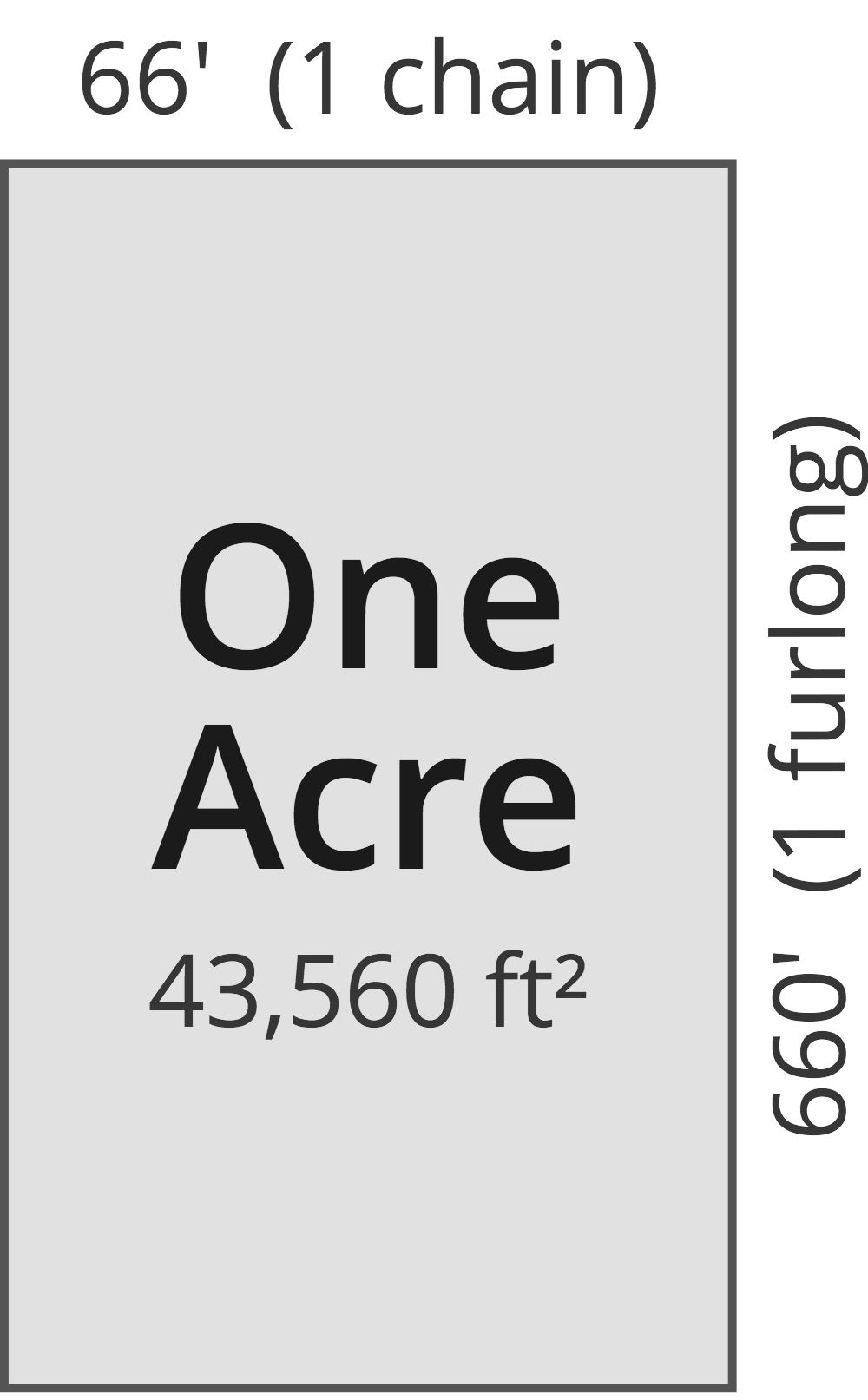# Acres to Square Miles Converter

Enter the area in acres below to get the value converted to square miles.

(find acres)
Results in Square Miles:1 ac = 0.001563 sq mi

Do you want to convert square miles to acres?

## How to Convert Acres to Square Miles

To convert a measurement in acres to a measurement in square miles, divide the area by the following conversion ratio: 640 acres/square mile.

Since one square mile is equal to 640 acres, you can use this simple formula to convert:

square miles = acres ÷ 640

The area in square miles is equal to the area in acres divided by 640.

For example, here's how to convert 500 acres to square miles using the formula above.
square miles = (500 ac ÷ 640) = 0.78125 sq mi## What Is an Acre?

One acre is defined as the area equal to a space that is one chain (66 ft) by one furlong (660 ft), or 10 square chains. That's equal to 43,560 square feet or 1/640 of a square mile for those unfamiliar with those units of measure.The acre is a US customary and imperial unit of area. Acres can be abbreviated as ac; for example, 1 acre can be written as 1 ac.

You can use an acreage calculator to measure the area of a plot of land in acres by locating the boundaries on a map.

## What Is a Square Mile?

One square mile is equal to the area of a square with sides that are each 1 mile long. One square mile is roughly equal to 2.59 square kilometers or 640 acres.

The square mile is a US customary and imperial unit of area. Square miles can be abbreviated as sq mi, and are also sometimes abbreviated as mi². For example, 1 square mile can be written as 1 sq mi or 1 mi².

## Acre to Square Mile Conversion Table

Table showing various acre measurements converted to square miles.
Acres Square Miles
1 ac 0.001563 sq mi
2 ac 0.003125 sq mi
3 ac 0.004688 sq mi
4 ac 0.00625 sq mi
5 ac 0.007813 sq mi
6 ac 0.009375 sq mi
7 ac 0.010938 sq mi
8 ac 0.0125 sq mi
9 ac 0.014063 sq mi
10 ac 0.015625 sq mi
20 ac 0.03125 sq mi
30 ac 0.046875 sq mi
40 ac 0.0625 sq mi
50 ac 0.078125 sq mi
60 ac 0.09375 sq mi
70 ac 0.109375 sq mi
80 ac 0.125 sq mi
90 ac 0.140625 sq mi
100 ac 0.15625 sq mi
200 ac 0.3125 sq mi
300 ac 0.46875 sq mi
400 ac 0.625 sq mi
500 ac 0.78125 sq mi
600 ac 0.9375 sq mi
700 ac 1.0938 sq mi
800 ac 1.25 sq mi
900 ac 1.4063 sq mi
1,000 ac 1.5625 sq mi

## References

1. National Institute of Standards and Technology, Specifications, Tolerances, and Other Technical Requirements for Weighing and Measuring Devices, Handbook 44 - 2019 Edition, https://nvlpubs.nist.gov/nistpubs/hb/2019/NIST.HB.44-2019.pdf